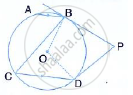Share

In the Given Figure, O is the Centre of the Circle. the Tangents at B and D Intersect Each Other at Point P. If Ab is Parallel to Cd and ∠Abc = 55°, Find: (I) ∠Bod (Ii) ∠Bpd - ICSE Class 10 - Mathematics

Question

In the given figure, O is the centre of the circle. The tangents at B and D intersect each other at point P. If AB is parallel to CD and ∠ABC = 55°, find:(i) ∠BOD (ii) ∠BPD

Solution

i)
∠BOD = 2 ∠BCD
⇒  ∠BOD =  2 × 55° =  110°
ii) Since, BPDO is cyclic quadrilateral, opposite angles are supplementary.
∴ ∠BOD  + ∠BPD  = 180°
⇒  ∠BPD =  180° -  110° =  70°

Is there an error in this question or solution?

APPEARS IN

Solution In the Given Figure, O is the Centre of the Circle. the Tangents at B and D Intersect Each Other at Point P. If Ab is Parallel to Cd and ∠Abc = 55°, Find: (I) ∠Bod (Ii) ∠Bpd Concept: Cyclic Properties.
S